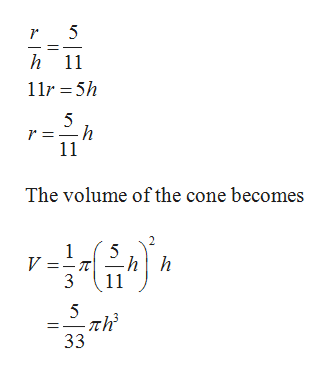# Suppose you are drinking root beer from a conical paper cup. The cup has a diameter of 10 centimeters and a depth of 11 centimeters. As you suck on the straw, root beer leaves the cup at the rate of 6 cm3/sec. At what rate is the level of the root beer in the cup changing when the root beer is 8 centimeters deep?The rate the root beer depth is dropping is (minus)  cm/sec.

Question
46 views

Suppose you are drinking root beer from a conical paper cup. The cup has a diameter of 10 centimeters and a depth of 11 centimeters. As you suck on the straw, root beer leaves the cup at the rate of 6 cm3/sec. At what rate is the level of the root beer in the cup changing when the root beer is 8 centimeters deep?

The rate the root beer depth is dropping is (minus)  cm/sec.

check_circle

Step 1

From the given information, the radius of the cone is 5 centimeters and height(depth) of the cone is 11 centimeters.

Step 2

Find the relation between r and h.help_outlineImage Transcriptioncloseh 11 11r 5h 5 -h r = 11 The volume of the cone becomes 2 1 V = 3 5 -hh 11 5 -л - πh 33 fullscreen
Step 3

Differentiate with respect to ...

### Want to see the full answer?

See Solution

#### Want to see this answer and more?

Solutions are written by subject experts who are available 24/7. Questions are typically answered within 1 hour.*

See Solution
*Response times may vary by subject and question.
Tagged in

### Calculus